Courses

# Computer Science And IT (CS/IT) Mock Test - 4 For Gate

## 65 Questions MCQ Test GATE Computer Science Engineering(CSE) 2022 Mock Test Series | Computer Science And IT (CS/IT) Mock Test - 4 For Gate

Description
This mock test of Computer Science And IT (CS/IT) Mock Test - 4 For Gate for GATE helps you for every GATE entrance exam. This contains 65 Multiple Choice Questions for GATE Computer Science And IT (CS/IT) Mock Test - 4 For Gate (mcq) to study with solutions a complete question bank. The solved questions answers in this Computer Science And IT (CS/IT) Mock Test - 4 For Gate quiz give you a good mix of easy questions and tough questions. GATE students definitely take this Computer Science And IT (CS/IT) Mock Test - 4 For Gate exercise for a better result in the exam. You can find other Computer Science And IT (CS/IT) Mock Test - 4 For Gate extra questions, long questions & short questions for GATE on EduRev as well by searching above.
QUESTION: 1

### Identify the correct spelling of the word.

Solution:

The correct spelling of the word is 'definitive' which means '(of a conclusion or agreement) done or reached decisively and with authority.' Thus option 2 is the correct answer.

QUESTION: 2

### This is the place that _______

Solution:

The preposition 'about' is mandatory here thus option 1 and 4 are eliminated. Option 2 is correct as the tense present perfect continuous fits here. It conveys the meaning that the person usually talked about the place.

QUESTION: 3

### She is brave. Her brother is more brave. Select the most suitable sentence with respect to grammar and usage.

Solution:

Options 1 and 2 are incorrect as they change the meaning of what is mentioned. Option 3 is incorrect as with 'less' we use the adjective in positive form and not comparative form. The correct word here should be 'brave.' Option 4 is thus the correct answer.

QUESTION: 4

When a four digit number is divided by 65, it leaves a remainder of 29. If the same number is divided by 13, the remainder would be______

Solution:

Let number is N

N = 65 k +29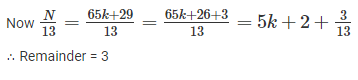QUESTION: 5

I was ___ ___  for the bus and then I ___ sight of Craig passing by.

Solution:

The word 'here' and 'there' both can be used here but should be followed with 'waiting' as no other word can fit here. The word 'caught' is correct here as 'catch a sight' means 'to see something.' Option 2 thus has the correct combination of words. The word 'cot' means 'a small bed with high barred sides for a baby or very young child.'

*Answer can only contain numeric values
QUESTION: 6

– digit number greater than 5000 are randomly formed from the digits 0, 2, 3, 5 and 7. The probability of forming a number divisible by 5 when the digits are repeated is ______

Solution:

For a number to be greater than 5000, d1 should be filled with either 5 or 7

∴ Total numbers formed when the digits are repeated = 2 × 5 × 5 × 5 = 250

total cases = 250 -1 = 249 ( case of 5000 is not included)

Now, For the number to be divisible by 5, unit digit d4 should be either 0 or 5.

∴ Total no. of ways = 2 × 5 × 5 × 2 = 100

favorable cases = 100 - 1=9 ( 5000 is not included))

∴Required Probability = 99/249 = 0.397

QUESTION: 7

It is theoretically possible that bacteria developed on Venus early in its history and that some were carried to Earth by a meteorite. However, strains of bacteria from different planets would probably have substantial differences in protein structure that would persist over time, and no two bacterial strains on Earth are different enough to have arisen on different planets. So, even if bacteria did arrive on Earth from Venus, they must have died out.

The argument is most vulnerable to which of the following criticisms?

Solution:

The question asks which of the statements given in the options can weaken the argument put by the author that all bacteria from Venus must have died out.

The passage states that since there is a single strain of bacteria which exists on the Earth, they all must be belonging to the Earth or let's say a single planet. But here the author does not take into consideration (as can be argued from his theory) the fact that may be all the bacteria came from Venus and there are none which originally belong to the Earth. So this criticism as mentioned in option 3 makes the argument of the author vulnerable.

Options 1, 2 and 4 are completely irrelevant criticisms as they do not address the main argument. The argument claims that if there were Venusian bacteria on Earth, then they must have died out by now. Whether there are bacteria originally from Earth that have also disappeared from Earth is irrelevant to the question and has no effect on the given argument.

QUESTION: 8

A man sells three articles A, B, C and gains 10% on A, 20% on B and loses 10% on C. He breaks even when combined selling prices of A and C are considered, whereas he gains 5% when combined selling prices of B and C are considered. What is his net loss or gain on the sale of all the articles?

Solution:

Let a, b and c be the cost prices of the three articles A, B and C.

SP = CP + Profit (or) SP = CP – Loss

⇒ SP of A = 1.1a; SP of B = 1.2b; SP of C = 0.9c

By question,

1.1a + 0.9c = a + c ⇒ 0.1a = 0.1c ⇒ a = c

1.2b + 0.9c = 1.05(b + c) ⇒ 0.15b = 0.15c ⇒ b = c = a

Gain% = {(SP – CP)/CP} × 100

Net gain on the sale of all the articles =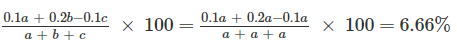Net gain on the sale of all the articles = 6.66%

QUESTION: 9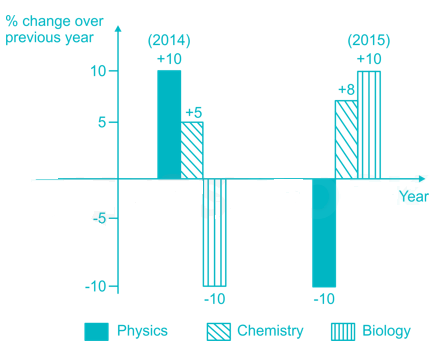Which of the following inferences can be drawn from the above graph?

Solution:

Option 1 is false as graph says there is decrease in students qualifying in Physics in 2015 compared to 2014.

Option 2

Let no. of students qualifying in Biology in 2013 be 100

⇒ No. of students qualifying in Biology in 2014 = 100 – 10% of 100 = 90

⇒ No. of students qualifying in Biology in 2015 = 90 + 10% of 100 = 99

∴ The number of students qualifying in Biology in 2015 is less than that in 2013

Option 3 and option 4 are incorrect since no detail is given regarding how many students qualified the subject in 2013.

QUESTION: 10

DRQP is a small square of side a in the corner of a big square ABCD of side A. What is the ratio of the area of the quadrilateral PBRQ to that of the square ABCD, given A/a = 3?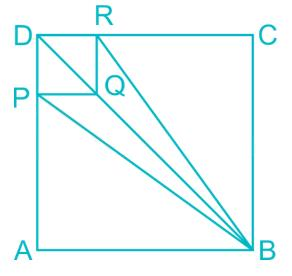Solution:

Area of triangle PAB = Area of triangle RCB (By symmetry)

∴ Area of Δ PAB = ½ × PA × AB

= ½ × 2A/3 × A = A2/3

Area of ΔRCB = A2/3

Now, Area of ABCD = Area of DRQP + Area of PAB + Area of RCB + Area of PBRQ

A2 = a2 + A2/3 + A2/3 + Area of PBRQ

As, A/a = 3 ⇒ a = A/3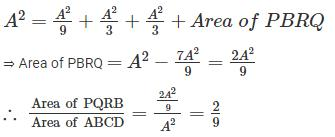QUESTION: 11

Given

Assume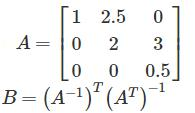Then the product of the eigen values of B is________

Solution: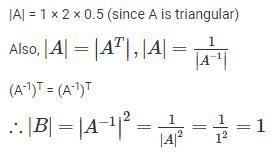QUESTION: 12

If 'k' is the number of states of the NFA, how many states will the DFA simulating NFA have?

Solution:

If 'k' is the number of states of the NFA, it has 2k subsets of states. Each subset corresponds to one of the possibilities that the DFA must remember, so the DFA simulating the NFA will have 2states.

QUESTION: 13

A complete graph is a graph in which each pair of graph vertices is connected by an edge. The chromatic number of a complete graph having 100 vertices is _______.

Solution:

The chromatic number of a complete graph is the number of vertices it has.

Therefore, the chromatic number of a complete graph having 100 vertices is 100.

*Answer can only contain numeric values
QUESTION: 14

A processor has 128 distinct instructions. A 24-bit instruction word has an opcode, register, and operand. The number of bits available for the operand field is 7. The maximum possible value of the general-purpose register is ______.

Solution:

No. of bits required for 128 instructions = log2 128 = 7

No. of bits required for operand field = 7

No. of bits required for register field = 24 – 7 – 7 = 10

Maximum no. of registers = 210 = 1024

i.e. from 0 to 1023. Hence, maximum value = 1023

*Answer can only contain numeric values
QUESTION: 15

The value of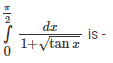Solution:

Let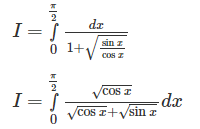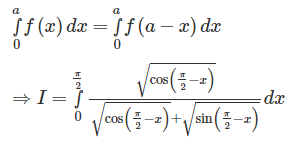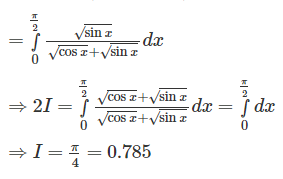QUESTION: 16

Which of the following expressions is equivalent to A.B+A′.B+A′.B′ ?

Solution:

The given expression can be solved as: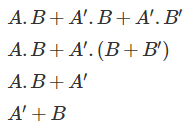*Answer can only contain numeric values
QUESTION: 17

Consider the following C function.

int strg(char *str)

{

static int temp=0;

if(*str!=NULL)

{

temp++;

strg(++str);

}

else

{

return temp;

}

}​

What is the output of strg(abcabcbb)?

Solution:
QUESTION: 18

The probability of a shooter hitting the target is 1/3 and three shots at the bull’s eye are needed to win the game. What could be the least number of shots for the shooter to give him more than half chance of winning the game?

Solution:

1 – P (x) ≥ 50%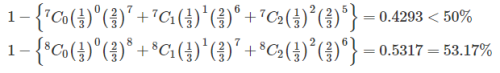QUESTION: 19

Suppose a circular queue of capacity n elements is implemented using an array. Circular queue uses REAR and FRONT as array index variables, respectively. Initially, REAR = FRONT = -1. The queue is initially full with 5 elements i.e. 1 2 3 4 5. After that 3 dequeue operations are performed. What is the condition to insert an element in to the above queue?

Solution:

In a circular queue, the new element is always inserted at the rear position. If queue is not full then, check if (rear == SIZE – 1 && front!= 0) if it is true then set rear=0 and insert new element.

QUESTION: 20

Which of the following is/are correct inorder traversal sequence(s) of binary search tree?

1. 5, 2, 6, 7, 9, 11, 1, 10

2. 10, 15, 16, 23, 38, 56, 89

3. 3, 7, 9, 16, 67, 88, 98

4. 7, 1, 8, 56, 34, 66, 45

Solution:

The inorder traversal of the binary search tree gives the sequence in ascending order.

Out of the given sequences, only 2 and 3 are in ascending order.

Therefore, option 2 is the correct answer.

*Answer can only contain numeric values
QUESTION: 21

Consider the propagation delay along the bus and through the ALU is 35 ns and 120 ns respectively. It takes 18 ns for a register to copy data from the bus. The total time required to transfer data from one register to another is ______ ns

Solution:

Transfer time = propagation delay + copy time

= 35 + 18

= 53 ns

QUESTION: 22

Consider the following set of statements:

S1: Given a context-free language, there is a Turing machine which will always halt in the finite amount of time and give answer whether language is ambiguous or not.

S2: Given a CFG and input alphabet, whether CFG will generate all possible strings of input alphabet (∑*) is undecidable.

S3: Consider three decision problems P1, P2 and P3. It is known that P1 is decidable and P2 is undecidable. P3 is undecidable if P2 is reducible to P3.

Which of the given statements is true?

Solution:

Statements S2 and S3 are true.

Statement S1 can be corrected as: Given a context-free language, there is no Turing machine which will always halt in the finite amount of time and give answer whether language is ambiguous or not.

QUESTION: 23

Consider a relation A with n elements. What is the total number of relations which can be formed on A which are irreflexive?

Solution:

A relation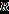on a setis irreflexive provided that no element is related to itself; in other words,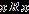for noin.

An irreflexive relation contains (n2 - n) elements.

Therefore, number of irreflexive relations =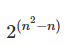QUESTION: 24

In the IPV4 addressing format, the 214 number of networks allowed under ______.

Solution:

In class B, size of net id = 16 bits

Size of host id = 16 bits

In network id first two bits are reserved for leading bits i.e. 10

Hence total number of networks possible = 216 – 2 = 214

*Answer can only contain numeric values
QUESTION: 25

What is the output of following C code?

#include <stdio.h>

#define MUL(x) (x * x)

int main( )

{

int i=4;

int p,q;

p= MUL(i++);

q = MUL(++i);

printf("%d", p + q);

return 0;

}

Solution:

In C, when preprocessor sees the #define directive, it goes through the entire program in search of the macro templates; wherever it finds one, it replaces the macro template with the appropriate macro expansion. In this program wherever the preprocessor finds the phrase MUL(x) it expands it into the statement (x * x).

p = 4 x 5 = 20

now i = 6

q = 8 x 8 = 64

p + q = 84

*Answer can only contain numeric values
QUESTION: 26

Consider a disk pack with 8 surfaces, 128 tracks per surface, 128 sectors per track and 512 bytes per sector. The number of bits required to address the sector is ______.

Solution:

Number of sectors  = Number of surfaces*Number of tracks per surface*Number of sectors per track

= 2* 27 * 27 = 217

Therefore, number of bits required = 17

QUESTION: 27

Consider the implementation of Dijkstra’s shortest path algorithm on a weighted graph G(V, E) with no negative edge. If this algorithm is implemented to find the shortest path between all pair of nodes, the complexity of the algorithm in a worst-case is ______.

Solution:

Dijkstra’s algorithm solves the single-source shortest-paths problem on a weighted, directed graph G for the case in which all edge weights are nonnegative. The time complexity of Dijkstra’s algorithm is O(E log V).

One can implement Dijkstra’s shortest path algorithm to find all pair shortest path by running it for every vertex. The time complexity of this Dijkstra’s algorithm is O(V3 log V).

QUESTION: 28

Consider the two cascaded 2-to-1 multiplexers as shown in the figure: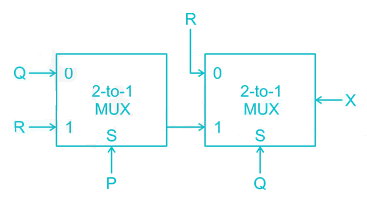The minimal sum of products form of the output X is

Solution:
*Answer can only contain numeric values
QUESTION: 29

In a TCP connection the size of the available buffer space in the receiver is 8 and senders window size is 2. The size of the congestion window is ________.

Solution:

A receiver and network can dictate to the sender the size of the sender's window. If the network cannot deliver the data as fast as they are created by the sender, it must tell the sender to slow down. In addition to the receiver, the network is a second entity that determines the size of the sender's window.

Actual window size = minimum (rwnd, cwnd)

rwnd = size of the receiver window

cwnd = size of the congestion window

2 = minimum(8, cwnd)

cwnd = 2

*Answer can only contain numeric values
QUESTION: 30

Consider two weighted complete graph G1 and G2 on the vertex set V1, V2, V3,…, V5 such that weight of the edge (Vi, Vj) is min(i,j) for the first graph and max(i,j) for the second graph respectively. The difference between the weight of a minimum spanning tree of G1 and G2 is ______.

Solution:
QUESTION: 31

A priority queue with n elements is implemented as a max heap. The time complexity to delete the element of the highest priority is ___________.

Solution:

Since the priority queue is implemented as a max heap, the maximum element will be present at the root node. Deletion of the element will lead to rebuilding the max heap.

Therefore, time complexity = O(log n).

QUESTION: 32

Consider the following C code:

struct emp

{

int empid;

char *name;

char *dept;

};

struct empDetails

{

int age;

char *city;

char *state;

struct emp employee;

};

int main()

{

Struct empDetails details;

…

}​

Which of the following syntax is the correct way to display employee name?

Solution:

A structure contains a number of data types grouped together. These data types may or may not be of the same type. Structure use a dot (.) operator to access its elements. One structure can be nested within another structure. The method used to access the element of a structure that is part of another structure. For this the dot operator is used twice.

*Answer can only contain numeric values
QUESTION: 33

Consider a system with byte-addressable memory, 40-bit logical address. What is the page size in MB if each page table entry is of 8 bytes each and size of page table is 8 MB?

Solution:

Page table size = number of page table entries x entry size

8 x 220= 240/k x 8

K = 240/220

K = 220= 1 MB

*Answer can only contain numeric values
QUESTION: 34

Study the following ER diagram carefully: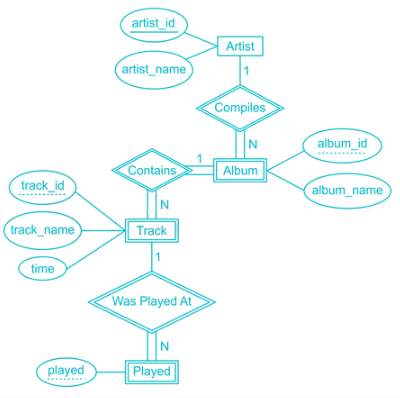How many total tables are required to store the data?

Solution:

Each strong and weak entity needs a separate table. Therefore, entities Artist, Album, Track and Played require 1 table each.

If the relationship is 1:n, we do not need any separate table to store it.

Therefore, total number of tables required = 4

QUESTION: 35

Say that string x is a prefix of string y if a string z exists where xz = y and that x is a proper prefix of y if in addition x ≠ y. Suppose an operation is defined on a regular language A. In which of the following options, the class of the regular language is closed?

Solution:

The language A is closed under NOPREFIX(A) = {ω Є A | no proper prefix of ω is a member of A}.

Let M = {Q, Ʃ, δ, q0, F} be an NFA recognizing A, where A is some regular language. Construct M' = {Q', Ʃ, δ', q0', F'} recognizing NOPREFIX(A) as:

Q' = Q

For r Є Q' and a Є Ʃ, define δ'(r, a)

δ'(r, a) = δ(r, a) if r ∉∉ F.

δ'(r, a) = ∅∅ if r Є F.

q0' = q0

F' = F

QUESTION: 36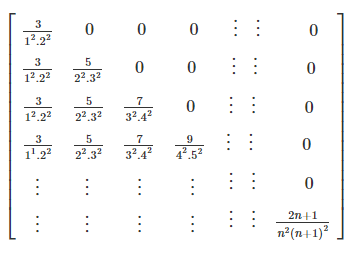Solution:

Sum of eigen value = trace of matrix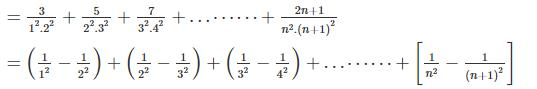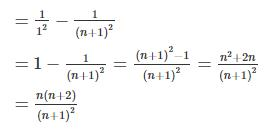*Answer can only contain numeric values
QUESTION: 37

A PC-relative mode branch instruction is 8 bytes long. The address of the instruction, in decimal, is 548321. Find the branch target address if the signed displacement in the instruction is –29.

Solution:

PC – relative mode branch instruction uses content of the program counter. Program counter points to the next instruction i.e. 548321 + 8 = 548329

Branch target address = 548329 – 29 = 548300.

*Answer can only contain numeric values
QUESTION: 38

TCP opens a connection using an initial sequence number of 3500 and sends data at 5 MBps. The other party opens the connection with a sequence number of 1200. Wrap around time for both the sequence number differs by 12562.77 sec. Calculate the data rate(in KB) for the second party.

Solution:

Wrap around time for the first sequence number: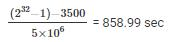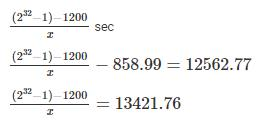X = 320000 Bytes per sec

X = 320 KB

QUESTION: 39

A gambler has 4 coins in his pocket. Two are double-headed, one is double-tailed, and one is normal. The coins cannot be distinguished unless one looks at them. The gambler takes a coin at random, opens her eyes and sees that the upper face of the coin is a head. What is the probability that the lower face is a head?

Solution:

Sample Space: {HH,HH,TT,TH/HT}

There are 5 faces that are heads out of a total 8, so probability is 5/8. Let A be the event that the upper face is a head, and B be the event that the lower face is head.

Pr[A] = Pr[B] = 5/8.

Pr[A∩B] = 2/4 = ½.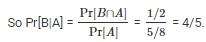Hence option b is correct.

*Answer can only contain numeric values
QUESTION: 40

Consider the minterm list form of a Boolean function F given below:

F(P, Q, R, S) = Ʃm(0, 1, 2, 5, 7,9, 10) + d(3, 8, 11, 14)

Here, m denotes a minterm and d denotes a don't care term. The number of essential prime implicants of the function F is ___________.

Solution: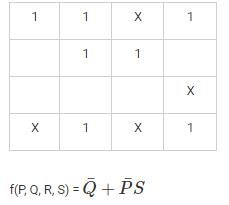QUESTION: 41

Consider the main memory with four page frames and the following sequence of page references:

11           3              5              9              6              5              3              6              5              11           8              9

Which one of the following page replacement policy experiences same no. of page hit?

I. FIFO

II. LRU

III. Optimal page replacement

IV. LIFO

Solution:
QUESTION: 42

Consider a DFS is implemented on an undirected weighted graph G. Let d(r,u) and d(r,v) be the weight of the edge (r,u) and edge (r, v) respectively. If v is visited immediately after u in depth first traversal, which of the following statement is correct?

Solution:

Depth-first search explores edges out of the most recently discovered vertex u that still has unexplored edges leaving it. Once all of u’s edges have been explored, the search “backtracks” to explore edges leaving the vertex from which u was discovered.

QUESTION: 43

Which of the following is equivalent to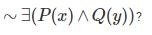Solution:

he correct answer is option 3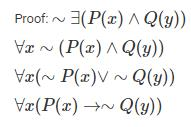QUESTION: 44

An operating system uses the banker's algorithm for deadlock avoidance to manage the allocation of four resources A, B, C, and D. The table given below represents the current system state.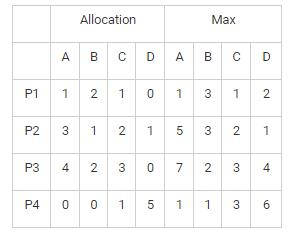There are 3 units of type B, 2 units of type D still available. The system is currently in the safe state. Which of the following sequence is a safe sequence?

Solution:

Available = {0, 3, 0, 2}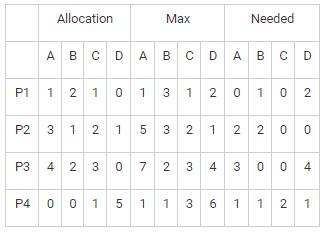QUESTION: 45

Which of the following statements is false?

Solution:

Option 3 is false.

If L1 and If L2 are two context free languages, their intersection L1 ∩ L2 need not be context free.

For example, L1 = { anbncm | n >= 0 and m >= 0 } and L2 = (ambncn | n >= 0 and m >= 0 }

L3 = L1 ∩ L2 = { anbncn | n >= 0 } need not be context free.

L1 says number of a’s should be equal to number of b’s and L2 says number of b’s should be equal to number of c’s. Their intersection says both conditions need to be true, but push down automata can compare only two. So it cannot be accepted by pushdown automata, hence not context free.

QUESTION: 46

Consider the following proposed solution for the two – process synchronization.

Code for P0:

do

{flag = true;

turn = 1;

while(flag && turn == 1);

(critical section)

flag = false;

(remainder section)

}

while(true);

Code for P1:

do

{flag = true;

turn = 0;

while(flag && turn == 0);

(critical section)

flag = false;

(remainder section)

}

while(true);

Above solution requires two shared data items: turn and flag[]

The variable turn indicates whose turn it is to enter its critical section. The flag array is used to indicate if a process is ready to enter its critical section.

Which of the following statement is TRUE?

Solution:

P0 and P1 could not have successfully executed their while statements at about the same time, since the value of turn can be either 0 or 1 but cannot be both. Hence, one of the processes say, P1 must have successfully executed the while statement, whereas P0 had to execute at least one additional statement. Hence mutual exclusion is preserved.

Once P1 exits its critical section, it will reset flag to false, allowing P0 to enter its critical section. If P1 resets flag to true, it must also set turn to 0. Thus, since P0 does not change the value of the variable turn while executing the while statement, P0 will enter the critical section (progress) after at most one entry by P1 (bounded waiting).

*Answer can only contain numeric values
QUESTION: 47

A bipartite graph is a set of graph vertices decomposed into two disjoint sets such that no two graph vertices within the same set are adjacent. What is the maximum number of edges in a bipartite graph having 6 vertices?

Solution:

There will be maximum edges if we have 3 (n/2) vertices in each set.

Therefore, maximum number of edges = (n/2)*(n/2) = n2/4 = 9

QUESTION: 48

What is the output of the following C code?

int main( )

{

auto int i=6;

{

auto int i=7;

{

auto int i=8;

printf ( "\n%d ", i ) ;

}

printf ( "%d ", i ) ;

}

printf ( "%d", i ) ;

}

Solution:

The Compiler treats the three i’s as totally different variables, since they are defined in different blocks. Once the control comes out of the innermost block the variable i with value 6 is lost, and hence the i in the second printf( ) refers to i with value 7. Similarly, when the control comes out of the next innermost block, the third printf( ) refers to the i with value 8.

QUESTION: 49

The characters ‘a’ to ‘e’ have the following frequencies. A Huffman code is used to represent the message. A message is made up of characters given below. What is the corresponding Huffman code for message ‘ace’?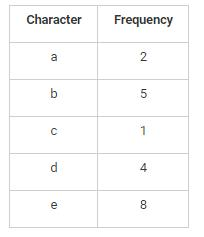Solution: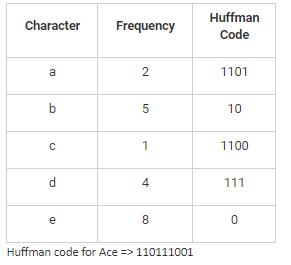QUESTION: 50

Consider the following set of statements:

S1: In a depth-first traversal of a graph G with n vertices, k edges are marked as tree edges. There are n-k connected components in G.

S2: A depth-first search necessarily finds the shortest path between the starting point and any other reachable node.

S3: The depth-first tree on the simple undirected graph never contains a cross edge.

Which of the given statements is false?

Solution:

Statement S2 is false. It can be corrected as:

A BFS will find the shortest path between the starting point and any other reachable node. A depth-first search will not necessarily find the shortest path.

*Answer can only contain numeric values
QUESTION: 51

In IP packet has arrived with datagram of size 700 bytes. The size of the IP header is 20 bytes. This packet will be forwarded on the link whose MTU is 185 bytes. The number of fragments that the IP datagram will be divided is ________.

Solution:

Maximum transmission unit = 185 bytes

Size of the IP header = 20 bytes

Number of fragments =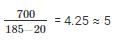QUESTION: 52

What is the generating function for the different ways in which eight identical cookies can be distributed among three distinct children if each child receives at least two cookies and no more than four cookies?

Solution:

Because each child receives at least two but no more than four cookies, for each child there is a factor equal to (x2 + x3 + x4) in the generating function for the sequence.

Because there are three children, this generating function is (x2 + x3 + x4)3

QUESTION: 53

The total number of number – tokens and literal – tokens in the following C code is ______.

int main()

{

float r, area;

printf("\nEnter the radius of Circle : ");

scanf("%d", &r);

area = 3.14 * r * r;

printf("\nArea of Circle : %f", area);

return 0;

}​

Solution: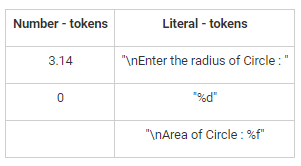QUESTION: 54

Which of the following problems is undecidable?

Solution:

Type-0 grammars include all formal grammars. Type 0 grammar language are recognized by turing machine. These languages are also known as the recursively enumerable languages.

The membership problem for Type-0 languages is undecidable.

*Answer can only contain numeric values
QUESTION: 55

A system has 3 resources and 5 processes competing for them. Each process can request a maximum of N instances. The largest value of N that will always avoid deadlock is _______.

Solution:

Distribute each process to one less than maximum request resources i.e. 5(N - 1)

Total no. of resources = 5(N - 1) + 1 = 5N - 2

If total no. resources are greater than given resources then a deadlock will occur.

5N – 2 <= 3

5N <= 5

N <= 1

*Answer can only contain numeric values
QUESTION: 56

The number of tables required to convert the relational schema R(A, B, C, D, E, F, G, H) into 3NF with following functional dependencies is _____.

A → DG

AB → E

D → C

E → F

G → H

Solution:

(AB)+ = {ABDGECFH}

Therefore, AB is the candidate key.

The given relation is in 1NF.

Converting in 2NF

R1(A, B, E, F) and R2(A, D, G, C, H)

Converting in 3NF

R11(A, B, E), R12(E, F)

R21(A, D, G), R22(D, C) and R23(G, H)

Hence, the number of tables is 5.

*Answer can only contain numeric values
QUESTION: 57

There are 6 stations in a slotted LAN. Probbability of each station transmits during a contention slot is 0.8. What is the probability of only one station transmits in a given time slot? (Compute the value upto 4 decimal places)

Solution:

The probability of only one station  =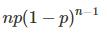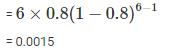QUESTION: 58

Consider the following relation:

Student(courseid, secid, semester, year, grade)

What is the expression for finding all the courses taught in the Fall 2009 semester but not in the Spring 2010 semester?

Solution:

The correct answer is option 3 i.e.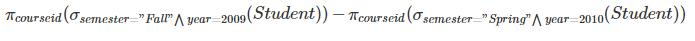QUESTION: 59

Consider the following parse tree for the expression 2^8 - 4 - 1 ^2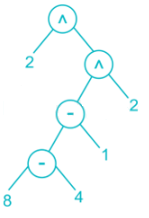The value of the given expression evaluated using the above parse tree is 512. Operator ^ is used to compute the power of a given number. What are the precedence order and associativity of the operator ^ and –?

Solution:

(2^(((8 – 4) – 1) ^2))

(2^((3^2)))

(2^9)

512

*Answer can only contain numeric values
QUESTION: 60

A cache memory unit with a capacity of 256 KB is implemented as a 4-way set-associative cache. What is the memory size (in MB) if number of tag bits is 6?

Solution:

Cache size = 256 KB = 218 B

Number of tag bits = 6

Memory is represented as: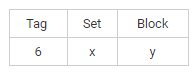Therefore, number of sets = 2x

Number of blocks = 2y

Cache size = Number of sets * Number of Lines per set * Block size

218 = 2* 4 * 2y

2x+y = 216

x+y = 16 bits

Therefore, width of physical address = 6 + 16 = 22 bits

Memory size = 222 = 4 MB

*Answer can only contain numeric values
QUESTION: 61

Let M1, M2, and M3 be three matrices of dimensions 12 x 9, 9 x 15, 15 x 10 respectively. The minimum number of scalar multiplications required to find the product M1 M2 M3 using the basic matrix multiplication method is ______.

Solution:

(M1 M2) M3:

12 x 9 x 9 x 15 -> resultant matrix: 12 x 15 -> no. of multiplications: 12 x 9 x 15 = 1620

12 x 15 x 15 x 10 -> resultant matrix: 12 x 10 -> no. of multiplications: 12 x 15 x 10 = 1800

Total no. of multiplication: 1620 + 1800 = 3420​

M1 (M2 M3):

9 x 15 x 15 x 10 -> resultant matrix: 9 x 10 -> no. of multiplications: 9 x 15 x 10 = 1350

12 x 9 x 9 x 10 -> resultant matrix: 12 x 10 -> no. of multiplications: 12 x 9 x 10 = 1080

Total no. of multiplication: 1350 + 1080 = 2430

QUESTION: 62

What is the output of following C code?

#include<stdio.h>

int main( )

{

char s[ ] = "C programming and Data structures" ;

printf ( "\n%s", &s ) ;

printf ( "\n%s", s ) ;

printf ( "\n%s", &s ) ;

printf ( "\n%c", s ) ;

return 0;

}

Solution: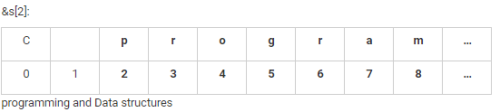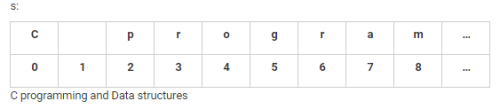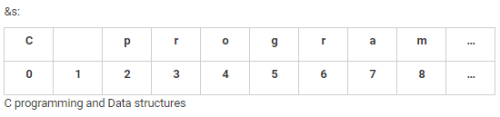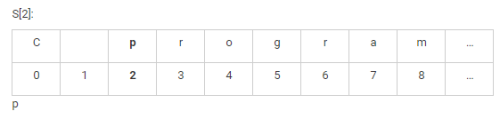*Answer can only contain numeric values
QUESTION: 63

A processor provides an instruction which transfers 64 bytes of data from one register to another register. Instruction fetch (IF) and Instruction decode (ID) takes 20 clock cycle. Then it takes 30 clock cycles to transfer each byte. The processor is clocked at a rate of 12 GHz. What is the delay in acknowledging an interrupt if the instruction is non-interruptible? (Compute value rounding to two decimal places.)

Solution:

Length of clock cycle = 1/12

Length of instruction cycle = (20 + (30 x 64)) x 1/12 = 161.67 ns

Worst case delay = length of instruction cycle = 161.67 ns

QUESTION: 64

Consider the following relations:

Student(ID, course, sec, semester, year)

Teacher(TID, course, sec, semester, year, salary)

What is the query to find the total number of (distinct) students who have taken courses taught by the instructor with ID 100?

Solution:

The correct answer is option 3 i.e.

select count (distinct ID)

from Student

where (course, sec, semester, year) in (select course, sec, semester, year

from Teacher where Teacher.TID= 100);

The IN operator is used when you want to compare a column with more than one value. It is similar to an OR condition.

*Answer can only contain numeric values
QUESTION: 65

Consider the slow start phase for a congestion control in a TCP connection. Initially, the window size is 4 MSS and the threshold is 36 MSS. At which transmission window size reached the threshold limit?

Solution:

Start ⇒⇒4 MSS

Window size after first transmission = 8 MSS

Window size after second transmission = 16 MSS

Window size after third transmission = 32 MSS

Window size after fourth transmission = 36 MSS